Question
 Be sure to answer all parts. Calculate the pH during the titration of 40.00 mL of 0.1000 M HCl with 0.1000 M NaOH solution after the following additions of base: a)28.00 ml b)39.40 ml c) 51.00 ml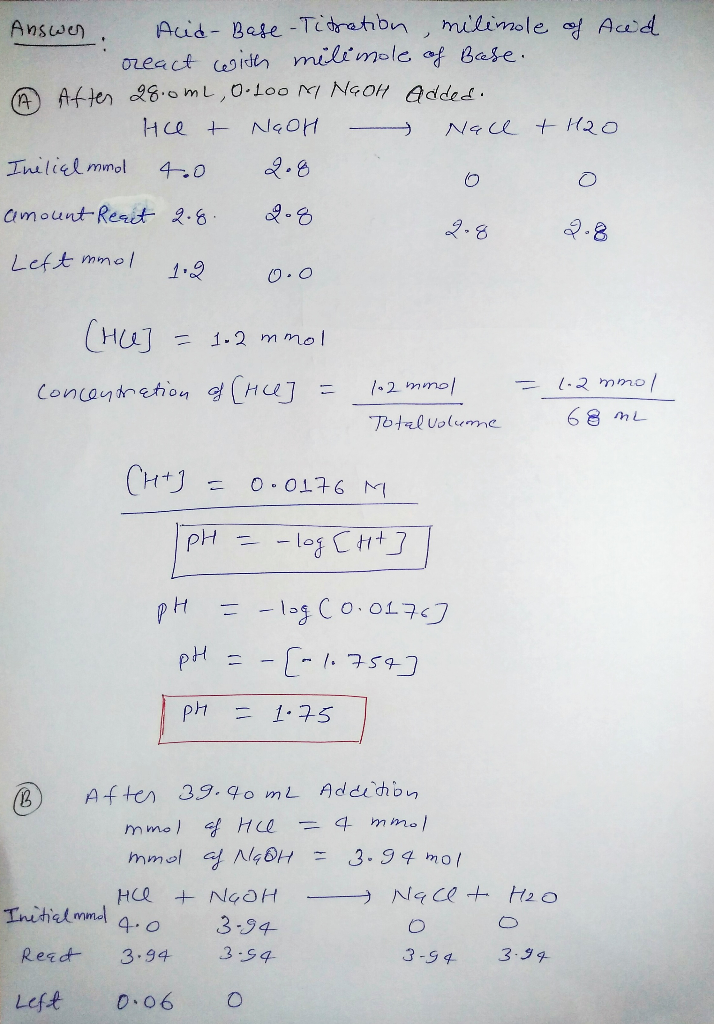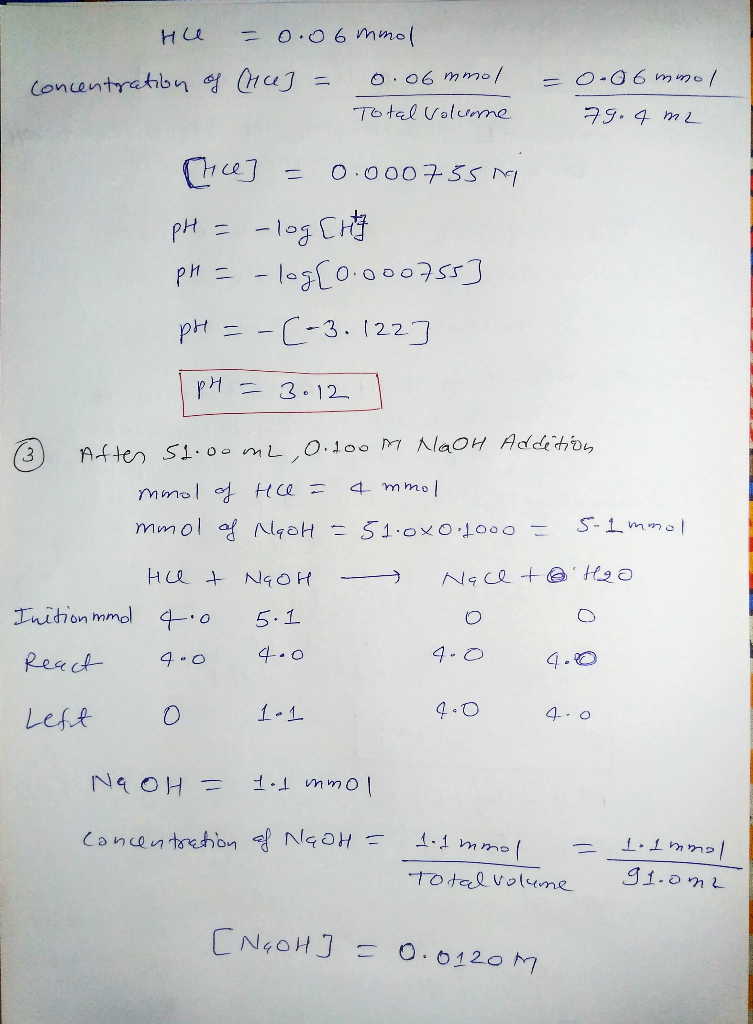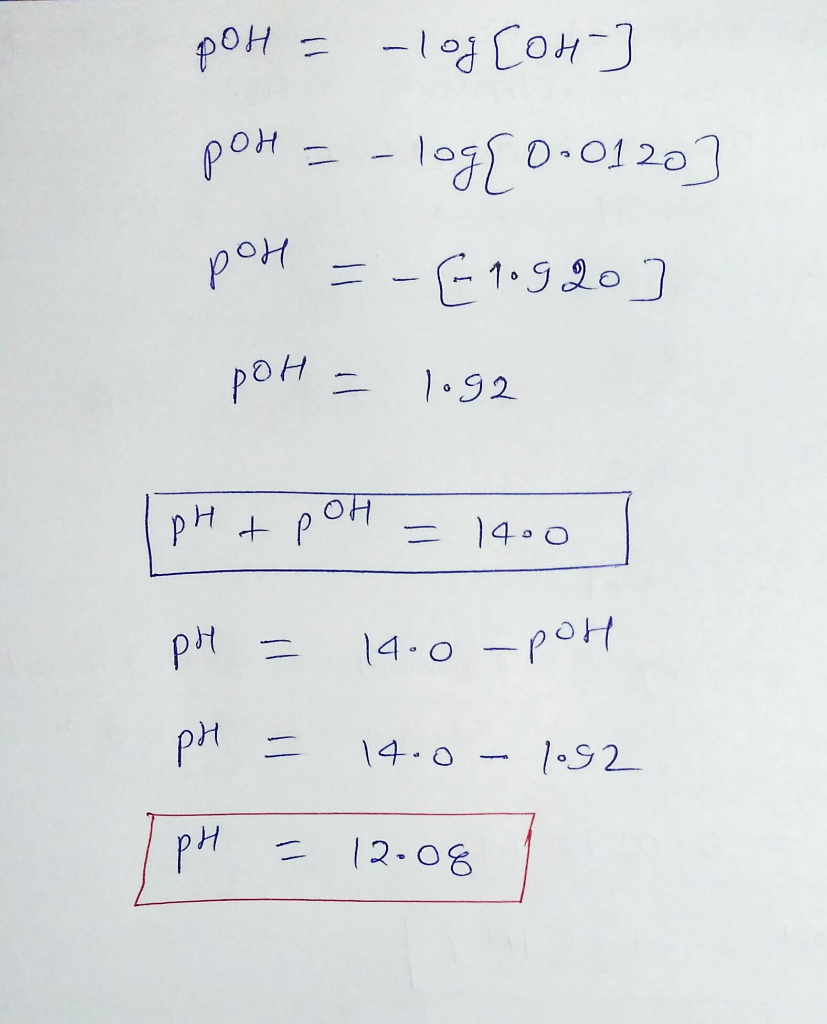#### Earn Coins

Coins can be redeemed for fabulous gifts.

Similar Homework Help Questions
• ### Be sure to answer all parts. Calculate the pH during the titration of 40.00 mL of...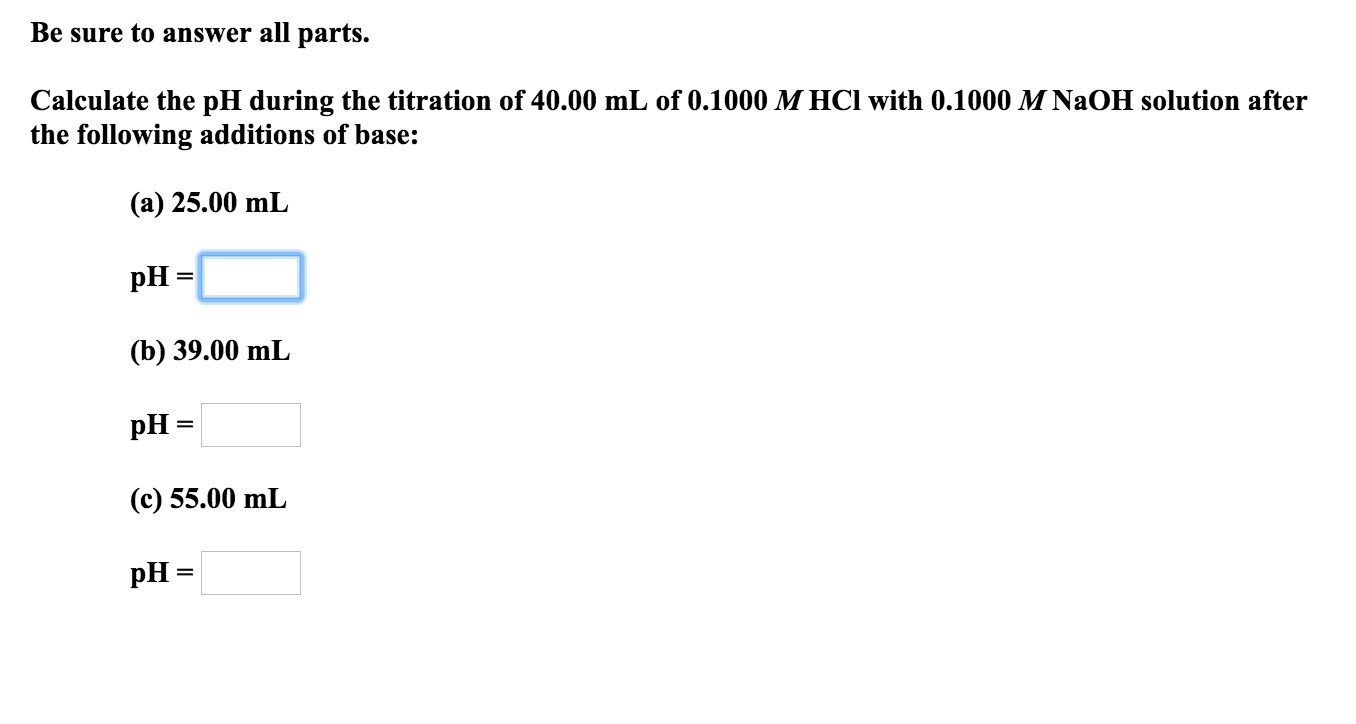Be sure to answer all parts. Calculate the pH during the titration of 40.00 mL of 0.1000 M HCl with 0.1000 M NaOH solution after the following additions of base: (а) 25.00 mL PН- (b) 39.00 mL pH (с) 55.00 mL pH

• ### steps needed with answers please Be sure to answer all parts. Calculate the pH during the...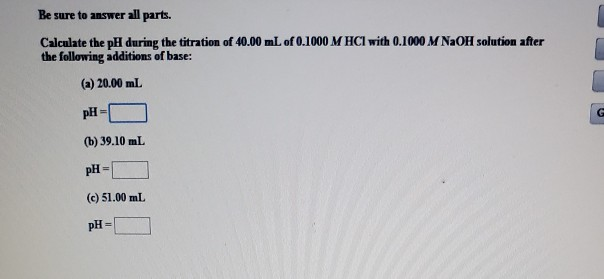steps needed with answers please Be sure to answer all parts. Calculate the pH during the titration of 40.00 mL of 0.1000 M HCl with 0.1000 M NaOH solution after the following additions of base: (a) 20.00 mL pH = (b) 39.10 mL pH = (c) 51.00 mL pH=

• ### Be sure to answer all parts. Find the pH during the titration of 20.00 mL of...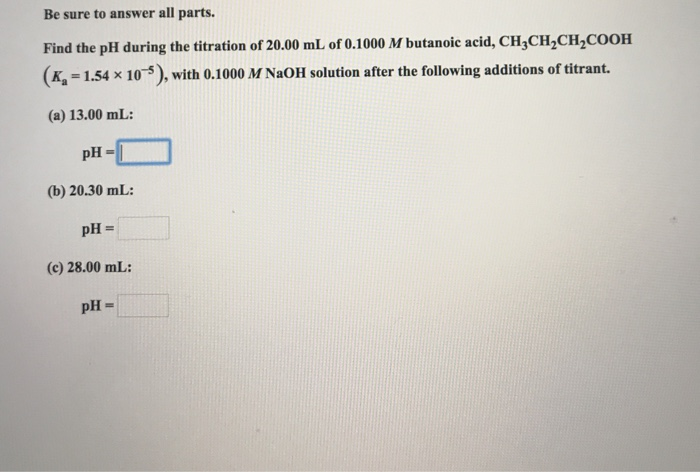Be sure to answer all parts. Find the pH during the titration of 20.00 mL of 0.1000 M butanoic acid, CHCH2CH2COOH (Ka = 1.54 x 10-5), with 0.1000 M NaOH solution after the following additions of titrant. (a) 13.00 mL: (b) 20.30 mL: (c) 28.00 mL:

• ### Be sure to answer all parts. Calculate the pH during the titration of 30.00 mL of...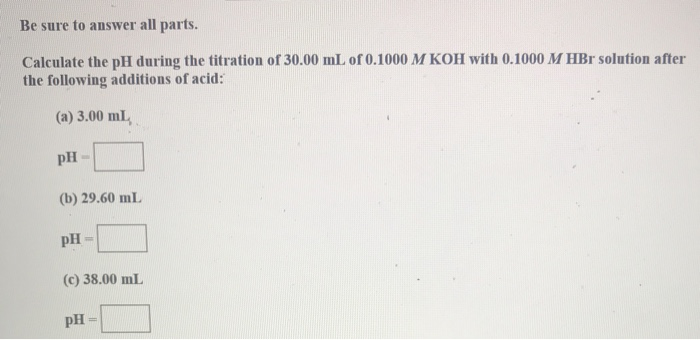Be sure to answer all parts. Calculate the pH during the titration of 30.00 mL of 0.1000 M KOH with 0.1000 M HBr solution after the following additions of acid: (a) 3.00 ml pH- (b) 29.60 mL pH = (C) 38.00 mL pH-

• ### Be sure to answer all parts. Calculate the pH during the titration of 30.00 mL of...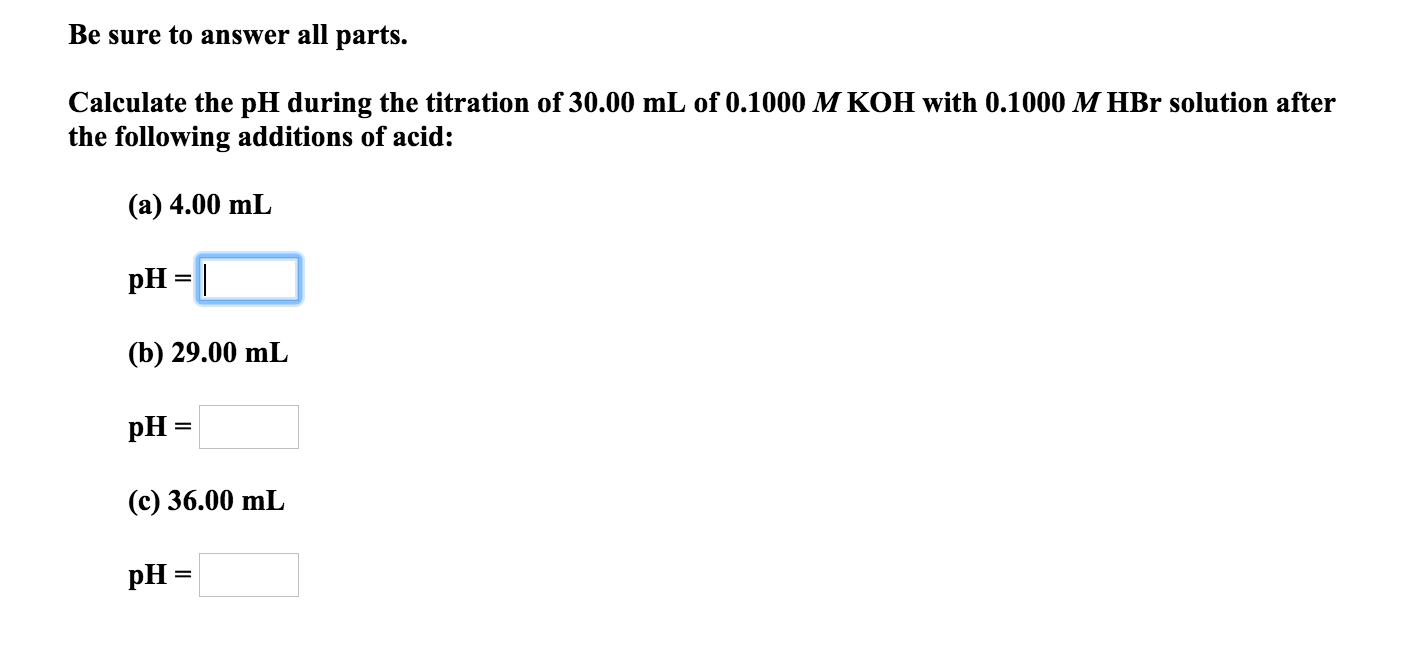Be sure to answer all parts. Calculate the pH during the titration of 30.00 mL of 0.1000 M KOH with 0.1000 M HBr solution after the following additions of acid: (a) 4.00 mL pH = (b) 29.00 mL pH = (c) 36.00 mL pH =

• ### Be sure to answer all parts. Find the pH during the titration of 20.00 mL of...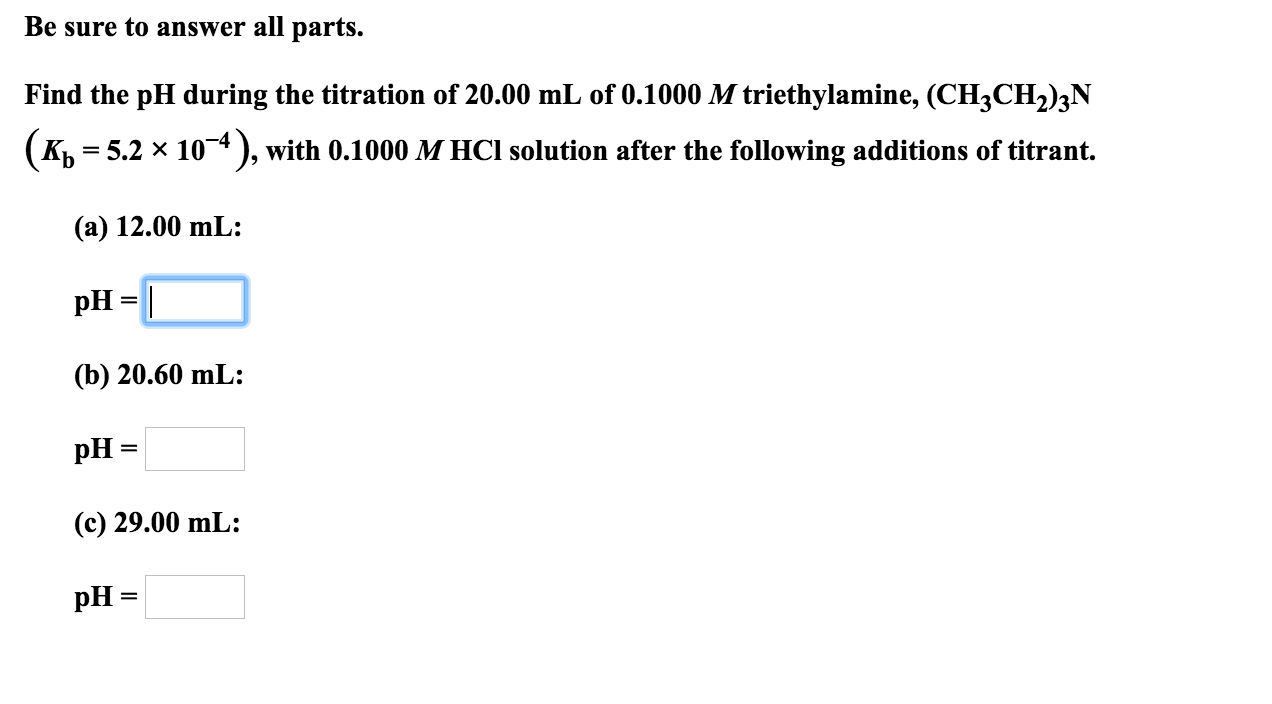Be sure to answer all parts. Find the pH during the titration of 20.00 mL of 0.1000 M triethylamine, (CH2CH2)2N (K) = 5.2 x 10-4), with 0.1000 M HCl solution after the following additions of titrant. (a) 12.00 mL: pH = 1 (b) 20.60 mL: pH = (c) 29.00 mL: pH =

• ### Be sure to answer all parts. Find the pH during the titration of 20.00 mL of...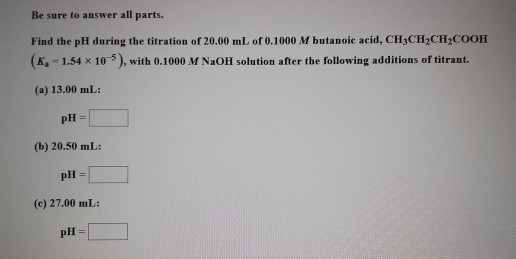Be sure to answer all parts. Find the pH during the titration of 20.00 mL of 0.1000 M butanoic acid, CH3CH2CH2COOH (K-1.54 x 10-5), with 0.1000 M NaOH solution after the following additions of titrant. (a) 13.00 mL: pH= (b) 20.50 mL: pH (c) 27.00 mL: pH=

• ### Be sure to answer all parts. Find the pH during the titration of 20.00 mL of...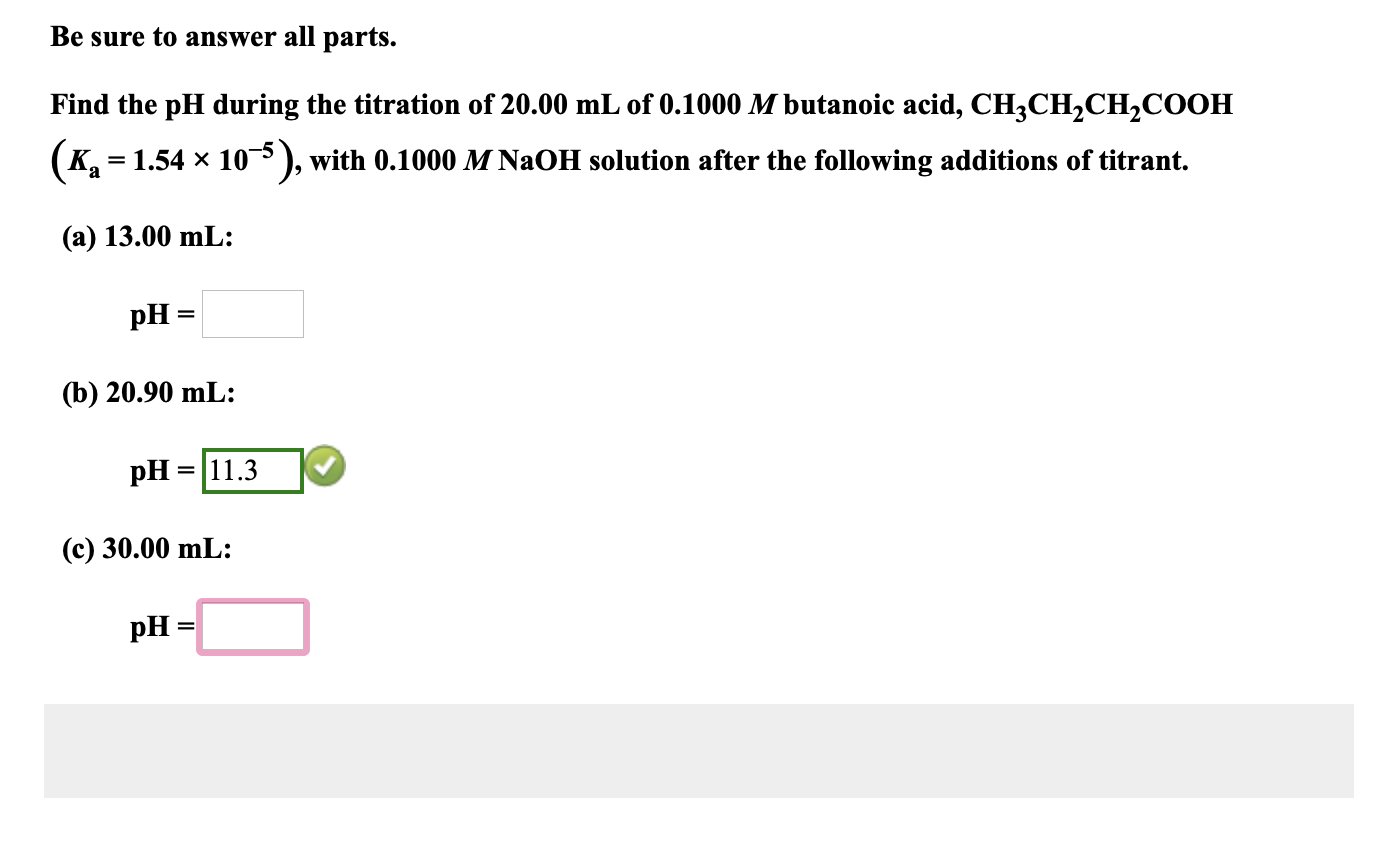Be sure to answer all parts. Find the pH during the titration of 20.00 mL of 0.1000 M butanoic acid, CH3CH2CH2COOH (K, = 1.54 x 10-5), with 0.1000 M NaOH solution after the following additions of titrant. (a) 13.00 mL: pH = (b) 20.90 mL: pH = 11. 30 (c) 30.00 mL: pH =

• ### Be sure to answer all parts. Find the pH during the titration of 20.00 mL of...

Be sure to answer all parts. Find the pH during the titration of 20.00 mL of 0.1000 M butanoic acid, CH3CH2CH2COOH (Ka = 1.54 × 10−5), with 0.1000 M NaOH solution after the following additions of titrant. A) 13.0 mL B) 20.20 mL C) 29.0 mL

• ### Be sure to answer all parts. Find the pH during the titration of 20.00 mL of...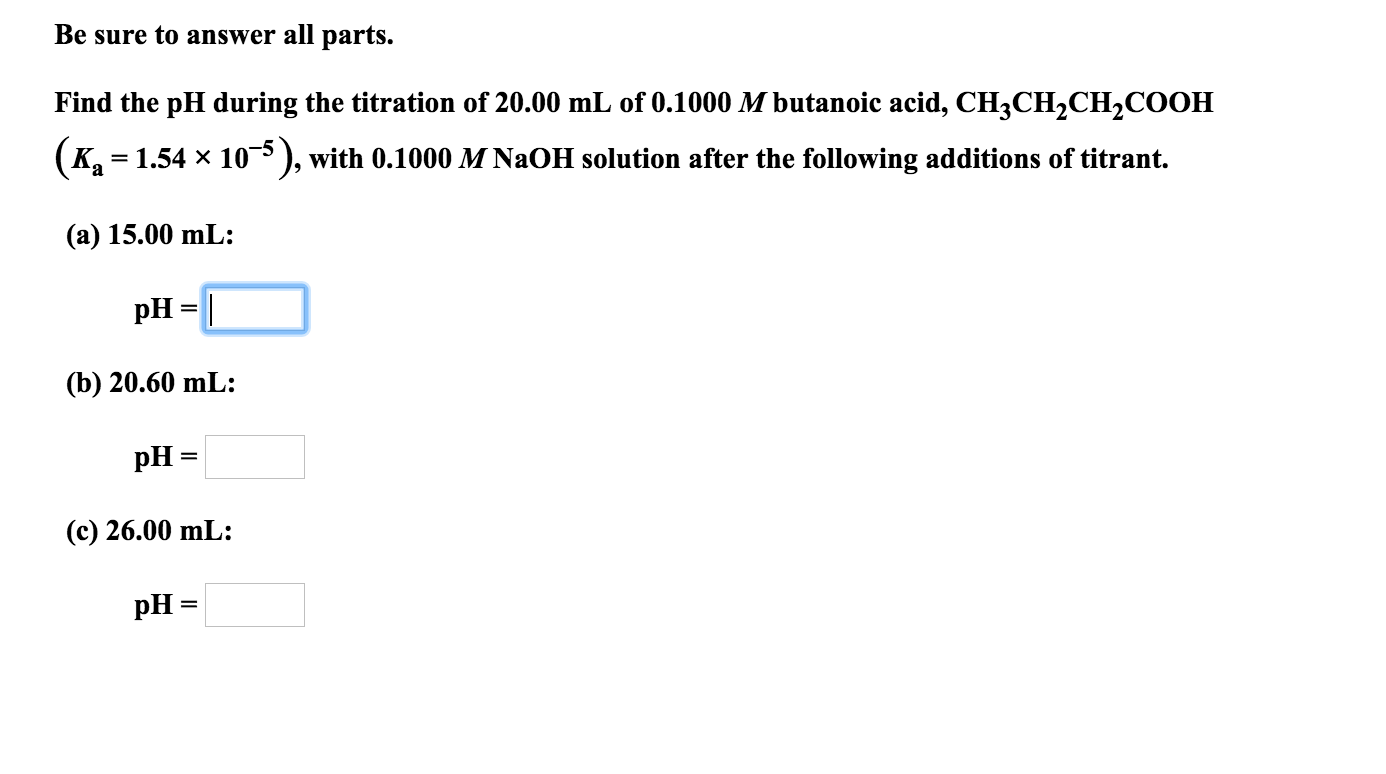Be sure to answer all parts. Find the pH during the titration of 20.00 mL of 0.1000 M butanoic acid, CH3CH2CH2COOH (K2 1.54 x 10, with 0.1000 M NaOH solution after the following additions of titrant. (а) 15.00 mL: pH (b) 20.60 mL: pH (с) 26.00 mL: pH